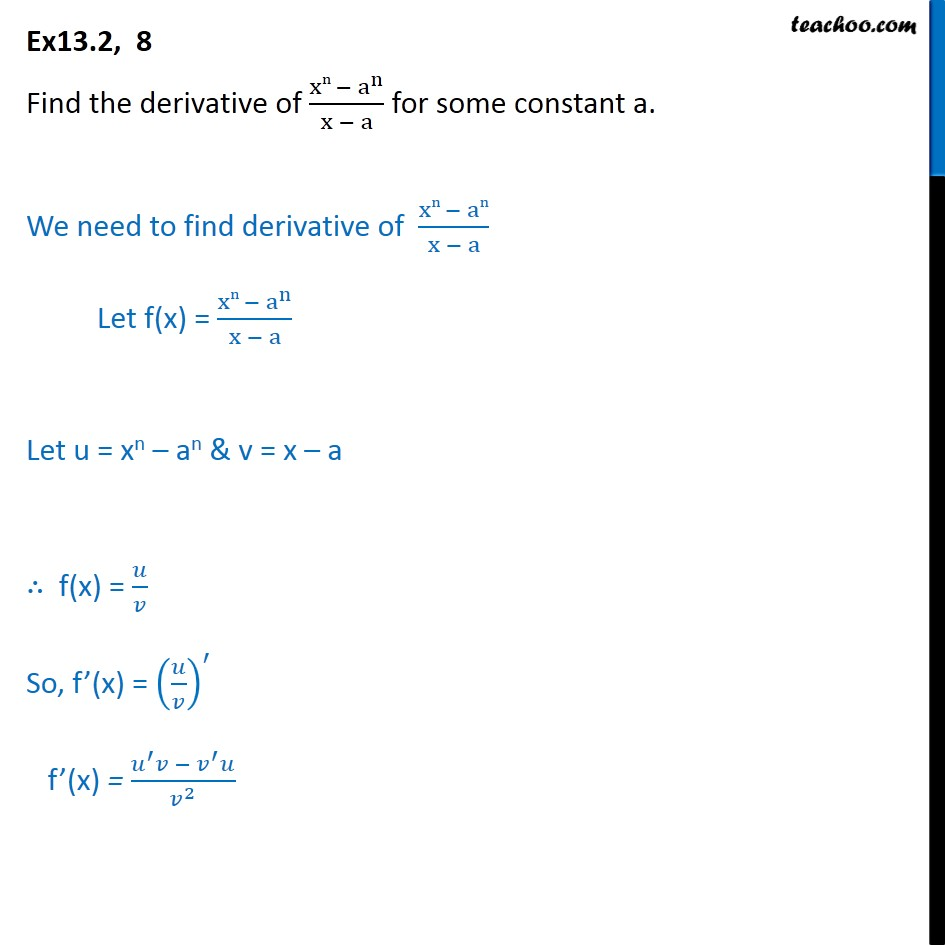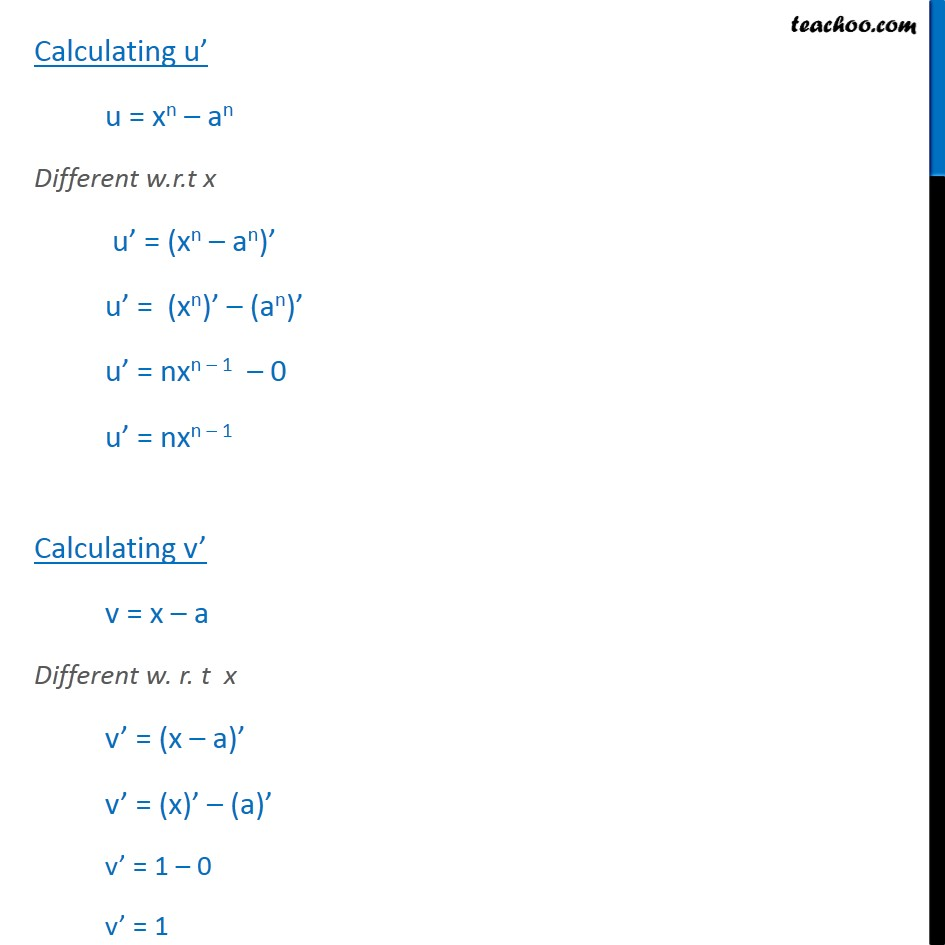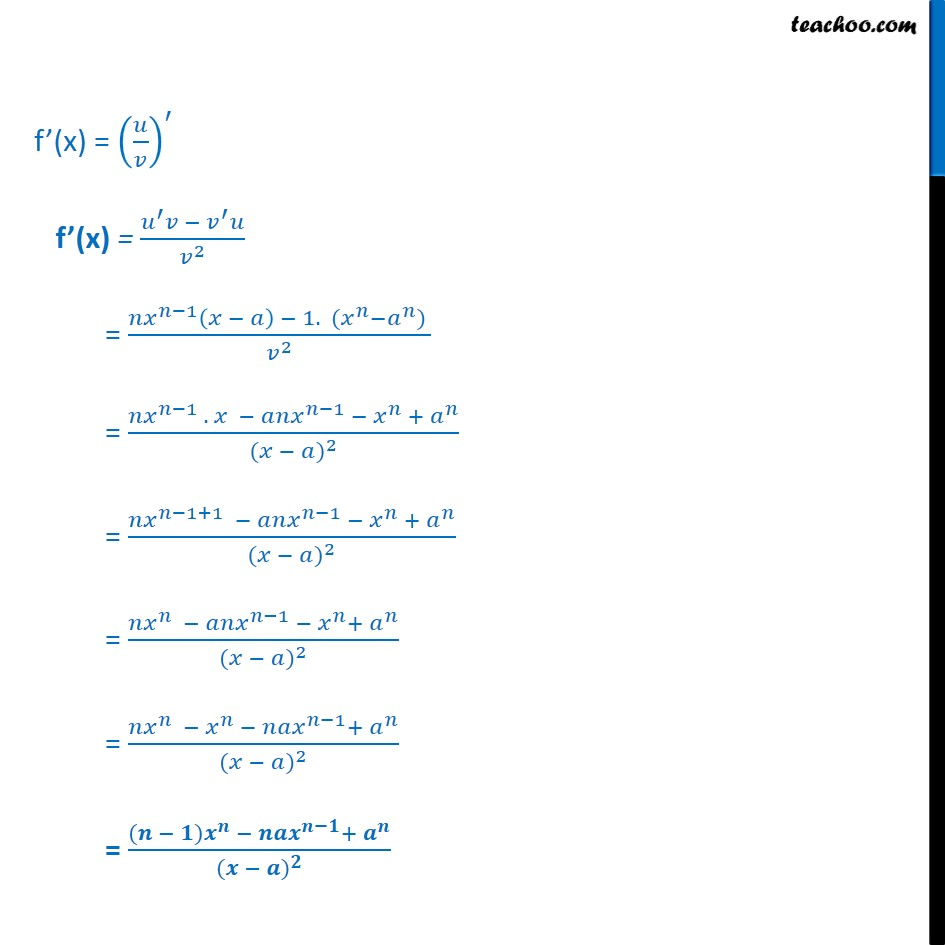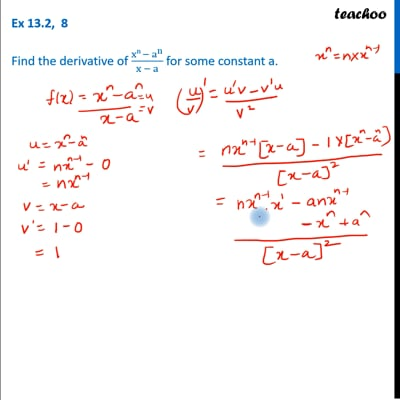Ex 13.2

Chapter 13 Class 11 Limits and Derivatives
Serial order wiseThis video is only available for Teachoo black users

Get live Maths 1-on-1 Classs - Class 6 to 12

### Transcript

Ex 13.2, 8 Find the derivative of xn a n x a for some constant a. We need to find derivative of xn an x a Let f(x) = xn a n x a Let u = xn an & v = x a f(x) = So, f (x) = f (x) = 2 Calculating u u = xn an Different w.r.t x u = (xn an) u = (xn) (an) u = nxn 1 0 u = nxn 1 Calculating v v = x a Different w. r. t x v = (x a) v = (x) (a) v = 1 0 v = 1 f (x) = f (x) = 2 = 1 1. ( ) 2 = 1 . 1 + ( ) 2 = 1+1 1 + ( ) 2 = 1 + ( ) 2 = 1 + ( ) 2 = ( ) + ( )